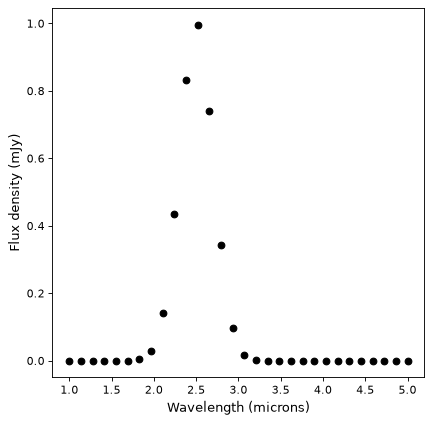# Support for units and quantities¶

Note

The functionality presented here was recently added. If you run into any issues, please don’t hesitate to open an issue in the issue tracker.

The astropy.modeling package includes partial support for the use of units and quantities in model parameters, models, and during fitting. At this time, only some of the built-in models (such as Gaussian1D) support units, but this will be extended in future to all models where this is appropriate.

## Setting parameters to quantities¶

Models can take Quantity objects as parameters:

>>> from astropy import units as u
>>> from astropy.modeling.models import Gaussian1D
>>> g1 = Gaussian1D(mean=3 * u.m, stddev=2 * u.cm, amplitude=3 * u.Jy)


Accessing the parameter then returns a Parameter object that contains the value and the unit:

>>> g1.mean
Parameter('mean', value=3.0, unit=m)


It is then possible to access the individual properties of the parameter:

>>> g1.mean.name
'mean'
>>> g1.mean.value
3.0
>>> g1.mean.unit
Unit("m")


If a parameter has been initialized as a Quantity, it should always be set to a quantity, but the units don’t have to be compatible with the initial ones:

>>> g1.mean = 3 * u.s
>>> g1
<Gaussian1D(amplitude=3. Jy, mean=3. s, stddev=2. cm)>


To change the value of a parameter and not the unit, simply set the value property:

>>> g1.mean.value = 2
>>> g1
<Gaussian1D(amplitude=3. Jy, mean=2. s, stddev=2. cm)>


Setting a parameter which was originally set to a quantity to a scalar doesn’t work because it’s ambiguous whether the user means to change just the value and preserve the unit, or get rid of the unit:

>>> g1.mean = 2
Traceback (most recent call last):
...
UnitsError : The 'mean' parameter should be given as a Quantity because it
was originally initialized as a Quantity


On the other hand, if a parameter previously defined without units is given a Quantity with a unit, this works because it is unambiguous:

>>> g2 = Gaussian1D(mean=3)
>>> g2.mean = 3 * u.m


In other words, once units are attached to a parameter, they can’t be removed due to ambiguous meaning.

## Evaluating models with quantities¶

Quantities can be passed to model during evaluation:

>>> g3 = Gaussian1D(mean=3 * u.m, stddev=5 * u.cm)
>>> g3(2.9 * u.m)
<Quantity 0.1353352832366122>
>>> g3(2.9 * u.s)
Traceback (most recent call last):
...
UnitsError : Units of input 'x', s (time), could not be converted to
required input units of m (length)


In this case, since the mean and standard deviation have units, the value passed during evaluation also needs units:

>>> g3(3)
Traceback (most recent call last):
...
UnitsError : Units of input 'x', (dimensionless), could not be converted to
required input units of m (length)


### Equivalencies¶

Equivalencies require special care - a Gaussian defined in frequency space is not a Gaussian in wavelength space for example. For this reason, we don’t allow equivalencies to be attached to the parameters themselves. Instead, we take the approach of converting the input data to the parameter space, and any equivalencies should be applied at evaluation time to the data (not the parameters).

Let’s consider a model that is Gaussian in wavelength space:

>>> g4 = Gaussian1D(mean=3 * u.micron, stddev=1 * u.micron, amplitude=3 * u.Jy)


By default, passing a frequency will not work:

>>> g4(1e2 * u.THz)
Traceback (most recent call last):
...
UnitsError : Units of input 'x', THz (frequency), could not be converted to
required input units of micron (length)


But you can pass a dictionary of equivalencies to the equivalencies argument (this needs to be a dictionary since some models can contain multiple inputs):

>>> g4(110 * u.THz, equivalencies={'x': u.spectral()})
<Quantity 2.888986819525229 Jy>


The key of the dictionary should be the name of the inputs according to:

>>> g4.inputs
('x',)


It is also possible to set default equivalencies for the input parameters using the input_units_equivalencies property:

>>> g4.input_units_equivalencies = {'x': u.spectral()}
>>> g4(110 * u.THz)
<Quantity 2.888986819525229 Jy>


## Fitting models with units to data¶

Fitting models with units to data with units should be seamless provided that the model supports fitting with units. To demonstrate this, we start off by generating synthetic data:

import numpy as np
from astropy import units as u
import matplotlib.pyplot as plt

x = np.linspace(1, 5, 30) * u.micron
y = np.exp(-0.5 * (x - 2.5 * u.micron)**2 / (200 * u.nm)**2) * u.mJy
plt.plot(x, y, 'ko')
plt.xlabel('Wavelength (microns)')
plt.ylabel('Flux density (mJy)')


(png, svg, pdf)and we then define the initial guess for the fitting and we carry out the fit as we would without any units:

from astropy.modeling import models, fitting

g5 = models.Gaussian1D(mean=3 * u.micron, stddev=1 * u.micron, amplitude=1 * u.Jy)

fitter = fitting.LevMarLSQFitter()

g5_fit = fitter(g5, x, y)

plt.plot(x, y, 'ko')
plt.plot(x, g5_fit(x), 'r-')
plt.xlabel('Wavelength (microns)')
plt.ylabel('Flux density (mJy)')


(png, svg, pdf)### Fitting with equivalencies¶

Let’s now consider the case where the data is not equivalent to those of the parameters, but they are convertible via equivalencies. In this case, the equivalencies can either be passed via a dictionary as shown higher up for the evaluation examples:

g6 = models.Gaussian1D(mean=110 * u.THz, stddev=10 * u.THz, amplitude=1 * u.Jy)

g6_fit = fitter(g6, x, y, equivalencies={'x': u.spectral()})

plt.plot(x, g6_fit(x, equivalencies={'x': u.spectral()}), 'b-')
plt.xlabel('Wavelength (microns)')
plt.ylabel('Flux density (mJy)')


(png, svg, pdf)In this case, the fit (in blue) is slightly worse, because a Gaussian in frequency space (blue) is not a Gaussian in wavelength space (red). As mentioned previously, you can also set input_units_equivalencies on the model itself to avoid having to pass extra arguments to the fitter:

g6.input_units_equivalencies = {'x': u.spectral()}
g6_fit = fitter(g6, x, y)